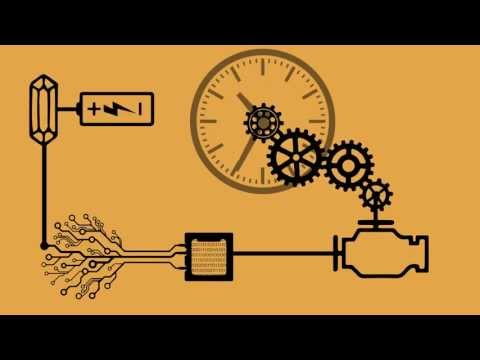## Measurement of Time

Subject: Science

#### Overview

The duration between any two events is called time. The SI unit of time is second. This note provides an information about the measurement of time.

#### Measurement of time

The duration between any two events is called time. The SI unit of time is second. In a pendulum clock, the oscillation is made for the measurement of time. One second is defined as the 1/86,400 th part of the mean solar day. A particular point in the space is called zenith. A solar day is defined as the time interval between the consecutive passages of the sun through the zenith.
The time taken by the earth to revolve once around the sun is called one year. Large distances such as the distance between the earth and the moon etc. are measured in the light year. It is the distance travelled by light in one year.

We have,

1 year = 365 days = 365 × 24 h = 365 × 24 × 3600 s = 31,536,000 s
Since the speed of the light in air or vacuum is 3× 108m/s
And distance travelled = speed × time
Therefore, 1 light year = 3 ×108× 31,536,000 s
= 9.4608 × 1015m

One standard second is defined as the time taken by cesium -133 atom to make 9192631770 vibrations. The watch based on such vibrations is called an atomic watch. Scientists use an atomic clock for the measurement of time.In quartz, watch time is measured by the oscillation of the pendulum and in an electronic watch, time is measured by frequency of alternating current. Automatic watch or mechanical watch may gain or lose time by few seconds or minutes in a day

Activity

You must have a clock in your home. Set the time of your clock according to the time of the news program of the radio. Then observe the time of your clock and news program of the radio after 1 month. What do you observe? Discuss with your friends and with your teachers.

##### Things to remember
• The duration between any two events is called time.
• The SI unit of time is second.
• One standard second is defined as the time taken by cesium -133 atom to make 9192631770 vibrations.
• One second is defined as the 1/86,400 th part of the mean solar day. A particular point in the space is called zenith.
• A solar day is defined as the time interval between the consecutive passages of the sun through the zenith.
• The time taken by the earth to revolve once around the sun is called one year.
• It includes every relationship which established among the people.
• There can be more than one community in a society. Community smaller than society.
• It is a network of social relationships which cannot see or touched.
• common interests and common objectives are not necessary for society.
##### Videos for Measurement of Time##### A Brief Introduction To The History Of Timekeeping Devices

The duration between any two events is called time. The SI unit of time is second.

One standard second is defined as the time taken by cesium -133 atom to make 9192631770 vibrations.

A solar day is defined as the time interval between the consecutive passages of the sun through the zenith.

One second is defined as the 1/86,400 th part of the mean solar day.

Solution:
We have,
1 year = 365 days = 365 × 24 h = 365 × 24 × 3600 s = 31,536,000 s
Since, the speed of the light in air or vacuum is 3× 108m/s
And distance traveled = speed × time
Therefore, 1 light year = 3 ×108× 31,536,000 s
= 9.4608 × 1015m

2 min

\begin{align*} \text {Converting into seconds} \\ &= 2\: \text {min} \\ &= 2\times 60\: \text {seconds} \:\:\: [\because 1  \:\text {min} = 60 \: \text {seconds}]\\ &= 120 \: \text {seconds} \\ \end{align*}

3 years

\begin{align*} \text {Converting into seconds} \\ &= 3\: \text {years} \\ &= 3\times 12\: \text {months} \:\:\: [\because 1 \: \text {year} = 12 \: \text {months}]\\ &= 36\: \text {months} \\ &= 36 \times 30\: \text {days} \: \:\:\: [\because 1\: \text {month} = 30 \: \text {days generally}] \\ &= 1080 \: \text {days} \\ &= 1080 \times 24 \: \text {hours} \:\:\: [\because 1\: \text {day} = 24 \: \text {hours}] \\ &= 25920 \: \text {hours} \\ &= 25920\times 60 \: \text {minutes} \:\:\: [\because 1\ \text {hour} = 60 \: \text {minutes}] \\ &=1555200 \:\text {minutes} \\ &=1555200 \times 60\:\:\: [\because 1\: \text {minute} = 60 \: \text {seconds}] \\ &= 93312000\: \text {seconds}\end{align*}

\begin{align*} 1 \: \text {mean solar day} &= 24 \: \text {hours} \:\:\: [\because 1\: \text {mean solar day} = 24\: \text {hours}] \\ &= 24 \: \text {hours} \\ &= 24 \times 60 \: \text {minutes} \: [\because 1 \: \text {hour} = 60 \: \text {minutes}]\\ &= 1440 \:\text {minutes} \\ &= 1440 \times 60\: \text {seconds}
\:\:\: [\because 1\: \text {minute} = 60 \: \text {seconds}] \\ = 86, 400 \: \text {seconds}\\\end{align*}